# Concept: Circles

INTRODUCTION

Circles is a very important topic for CAT and other MBA entrance exams. A variety of questions can be asked from this topic standalone or in combination with other geometry topics.

A circle is a set of all points in a plane that lie at a constant distance from a fixed point. The fixed point is called the center of the circle and the constant distance is known as the radius of the circle.

BASIC TERMS

A radius is the distance between center of the circle and any point on the circle.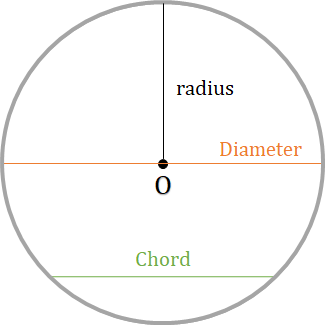​​​​​​​

Chord
A chord is a line segment within a circle that touches 2 points on the circle.

Diameter (d)
The longest distance from one end of a circles to the other is known as the diameter. It is equal to twice the radius and passes through the center.

Circumference (C)
The perimeter of the circle is called the circumference. The value of the circumference = 2πr, where r is the radius of the circle.

Area (A)
Area of a circle = πr2.

Sector
A sector is like a slice of pie (a circular wedge). [The green shaded portion in the figure below is a sector]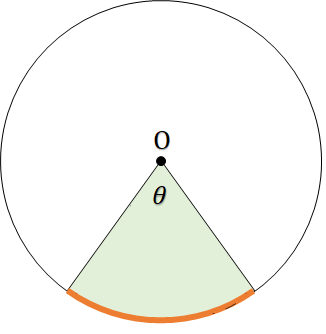​​​​​​​

Area of a sector (with central angle 𝜃) = $\frac{𝜃}{360°}$ × πr2

Arc
An arc is a curved line that is part of the circumference of a circle. A minor arc is an arc less than the semicircle and a major arc is an arc greater than the semicircle. [Orange strip in the figure above is the minor arc.]

Length of Arc (with central angle 𝜃) = $\frac{𝜃}{360°}$ × 2πr

Secant
A line that intersects two points on the circle.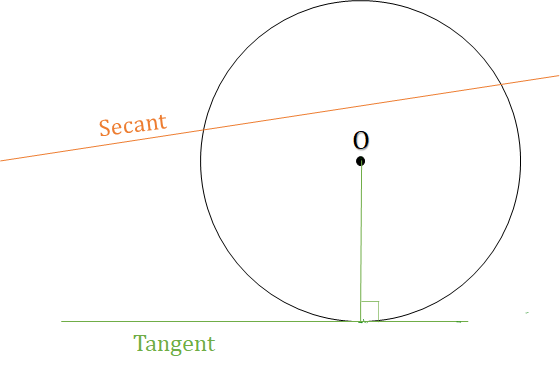​​​​​​​

Tangent
A line that touches only one point on the circle and is ⊥ to the radius.

Segment
A chord divides the circle in two parts called segments. The smaller region is called the minor segment while the larger region is called the major segment.[Green shaded region in the figure below is the major segment while the orange shaded region is minor segment.]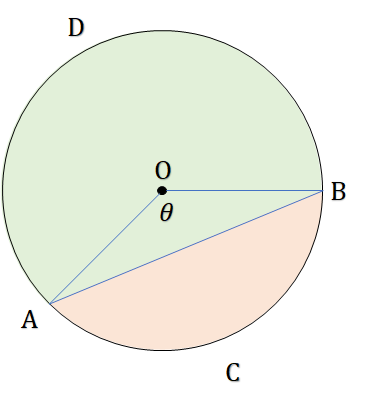​​​​​​​

Area of minor segment ACB (with central angle 𝜃) = $\frac{𝜃}{360°}$ × πr2 - $\frac{1}{2}$ × r2 × 𝑆𝑖𝑛𝜃

Angle subtended by an arc on the circle in the same segment
Here, the angle subtended by arc ADB in the opposite segment is 𝛼° and in the same segment is (180 - 𝛼)°.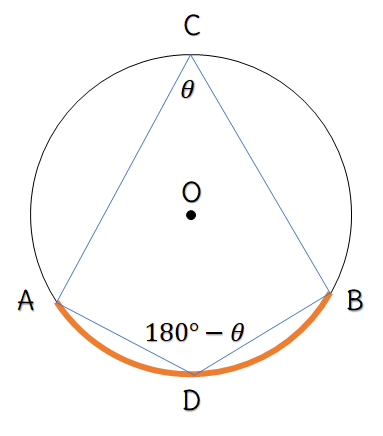​​​​​​​

PROPERTIES OF CIRCLES

Perpendicular from Center Bisects the Chord

The perpendicular from the center of a circle to a chord of the circle bisects the chord. In the figure below, O is the center of the circle and OM ⊥ AB. Then, AM = MB.​​​​​​​

Conversely, the line joining the centre of the circle and the midpoint of a chord is perpendicular to the chord.

Equal chords are equidistant from the center

Equal chords of a circle are equidistant from the center.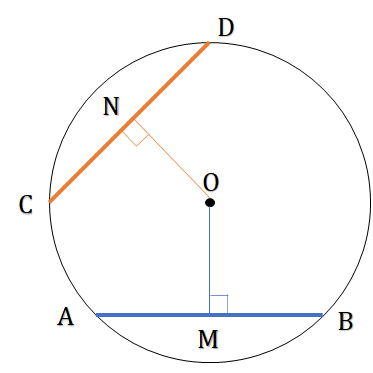​​​​​​​

Conversely, if two chords are equidistant from the center of a circle, they are equal in length.

Also, equal chords subtend equal angles at the center.

Angle subtended by an Arc at center

The angle subtended by an arc of a circle at the center is double the angle subtended by it at any point on the remaining part of the circumference.​​​​​​​

Angle subtended by an Arc on circle

The angles subtended by an arc of a circle in same segment are equal.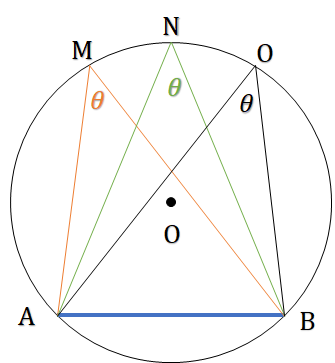​​​​​​​

Angle subtended by an Arc in alternate segments

Angles subtended by a chord at distinct points on circumference, in alternate segments are supplementary.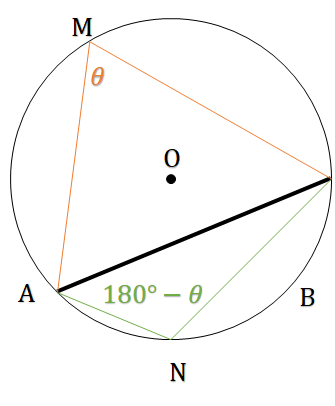​​​​​​​

Two intersecting Chords

In the following figure, two chords of a circle, AB and CD, intersect at point P. Then AP × PB = CP × PD.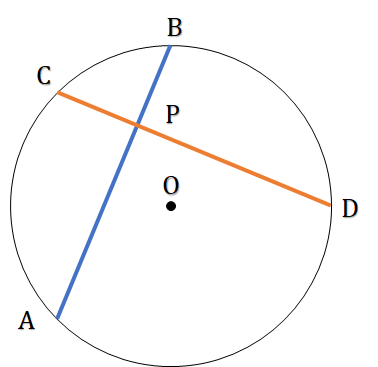​​​​​​​

Angle subtended by the Diameter

An angle inscribed in a semi-circle is a right angle. In other words, angle made by diameter anywhere on the circle is 90°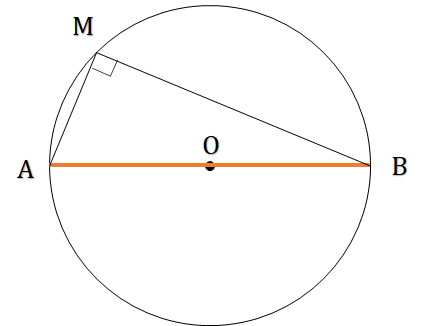​​​​​​​

If a straight line is tangent to a circle, then the radius drawn to the point of contact will be perpendicular to the tangent.​​​​​​​

Two tangents drawn from an external point

If two tangents are drawn to a circle from an exterior point, the length of two tangent segments are equal. Also, the line joining the exterior point to the center of the circle bisects the angle between the tangents.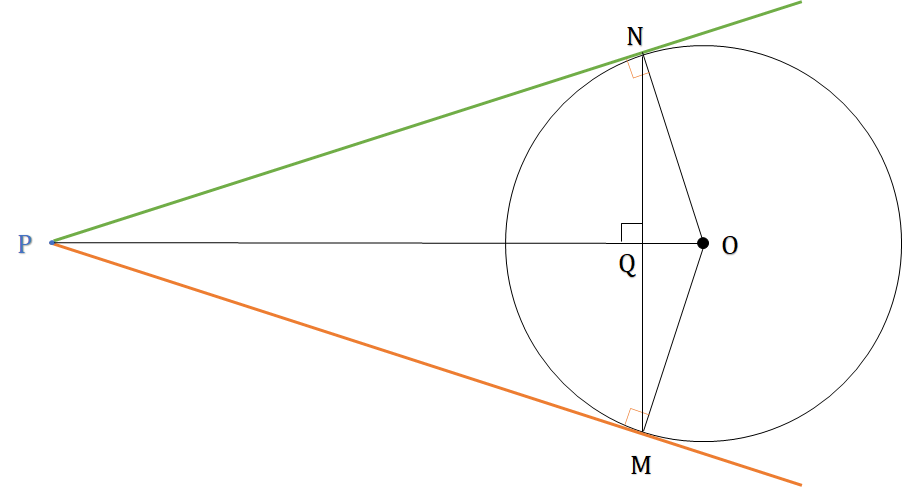​​​​​​​

Here,
∆PON ≈ ∆POM
PN = PM
MN ⊥ OP
∠NPO = ∠MPO = ∠QNO = ∠QMO
∠NOP = ∠MOP = ∠PNQ = ∠PMQ

2 Secants drawn from external point

From an external point P, two secants PAB & PMN are drawn. Then, PA × PB = PM × PN​​​​​​​

Tangent Secant Theorem

From an external point P, a secant PAB, intersecting the circle at A and B, and a tangent PC are drawn. Then, PA × PB = PT2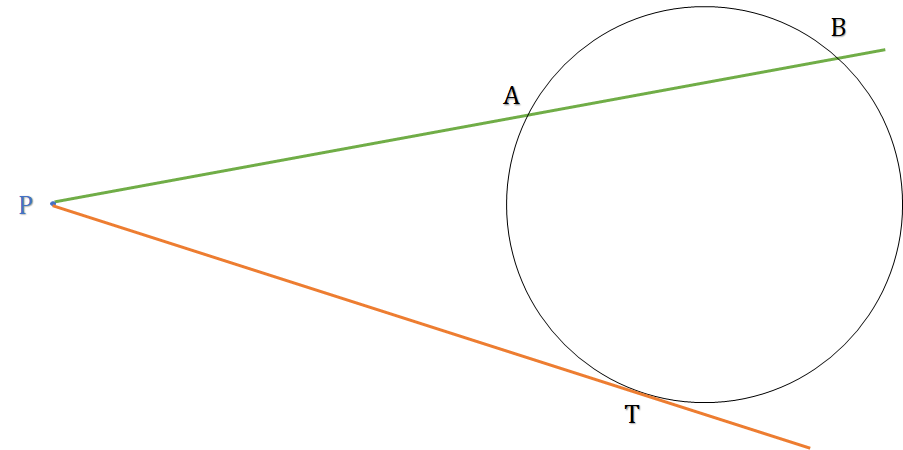​​​​​​​

Alternate Segment Theorem

The angle that a tangent to a circle makes with a chord drawn from the point of contact is equal to the angle subtended by that chord in the alternate segment of the circle.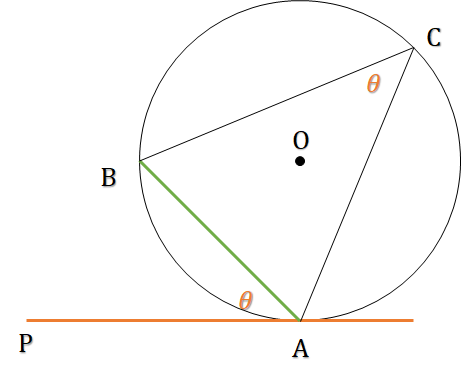​​​​​​​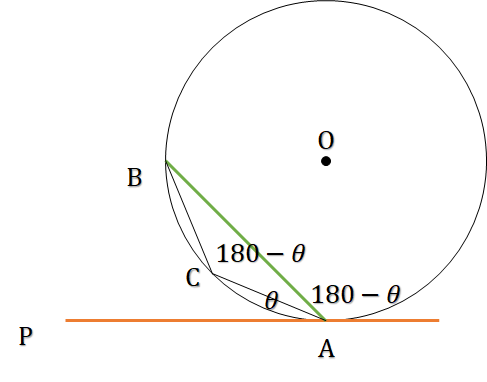​​​​​​​

Circumcircle and Incircle

Circumcircle: Circle circumscribes the quadrilateral, or Quadrilateral is circumscribed by the circle.​​​​​​​

Incircle: Quadrilateral circumscribes the circle, or Circle is circumscribed by the quadrilateral.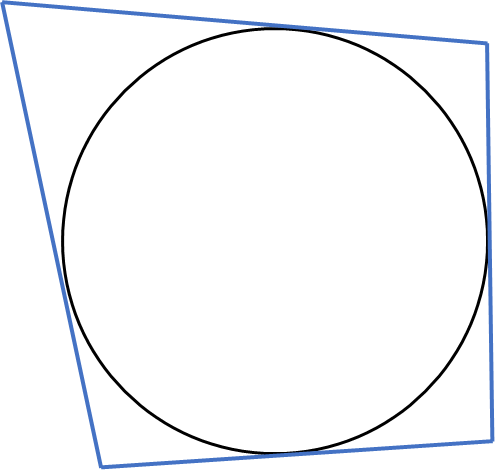​​​​​​​

A quadrilateral whose all 4 vertices lie on the same circle is called a cyclic quadrilateral. The sum of any two opposite angles of a cyclic quadrilateral is 180°.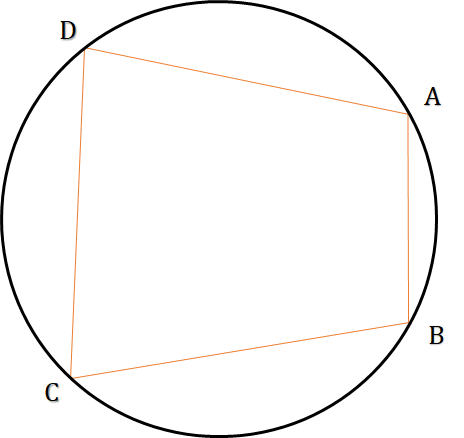​​​​​​​

Ptolemy's Theorem

In a cyclic quadrilateral PQRS, the product of diagonals is equal to the sum of the products of the length of the opposite sides.
⇒ AC × BD = AB × CD + BC × AD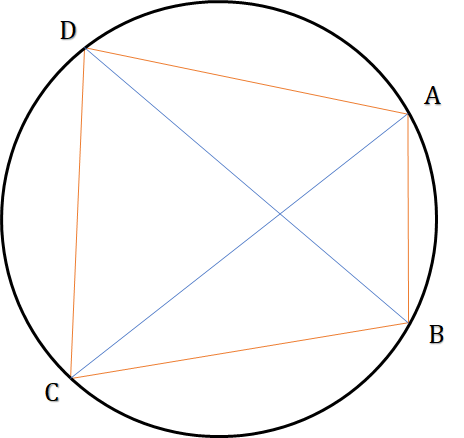​​​​​​​

Cyclic Trapezium

In a cyclic trapezium, the two non-parallel sides are equal and the angles opposite them are also equal, i.e., it is an isosceles trapezium​​​​​​​

Conversely, an isosceles trapezium will always be a cyclic trapezium

Note: If a quadrilateral circumscribes a circle, then sum of opposite sides are equal.
⇒ AB + CD = AD + BC​​​​​​​

Two Circles

Two circles intersecting

When two circles intersect each other, the line joining the centers bisects the common chord and is perpendicular to the common chord.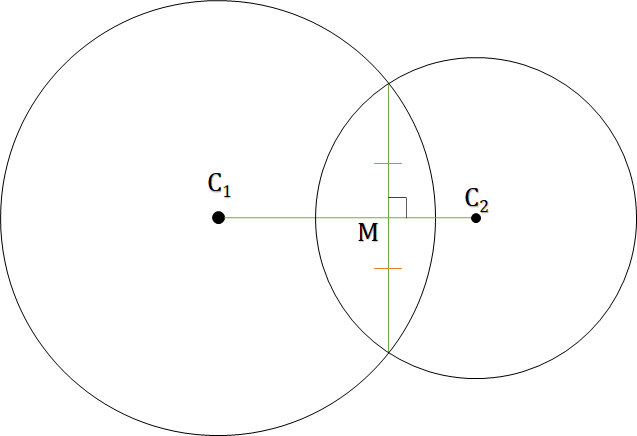​​​​​​​

Two circles touch externally

When two circles touch each other externally at a point P, the line joining the centers passes through this point P.​​​​​​​

Common Tangents of two Circles

When two circles intersect / touch the number of common tangents will be

4 if they do not touch each other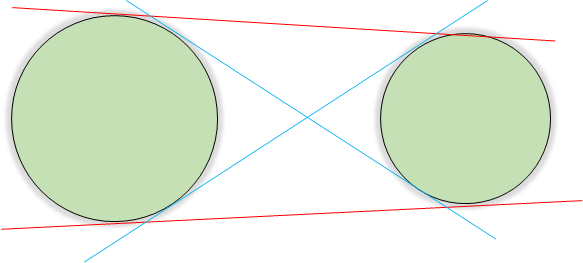​​​​​​​

3 if they touch each other externally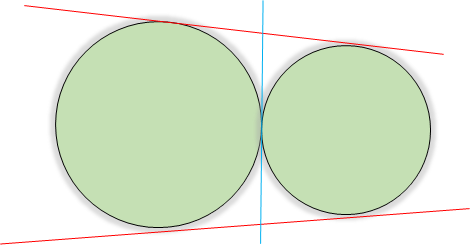​​​​​​​

2 if they intersect at exactly two different points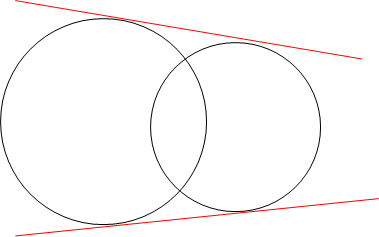​​​​​​​

1 if they touch each other internally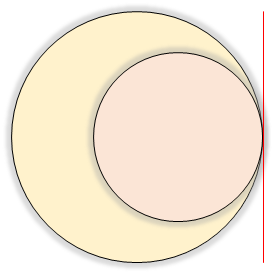​​​​​​​

0 if the the smaller circle is completely inside the larger circle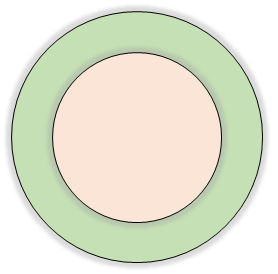​​​​​​​

Length of Tangents

Direct Common Tangent (DCT) = $\sqrt{{d}^{2}-{\left({r}_{1}-{r}_{2}\right)}^{2}}$

Transverse Common Tangent (TCT) = $\sqrt{{d}^{2}-{\left({r}_{1}+{r}_{2}\right)}^{2}}$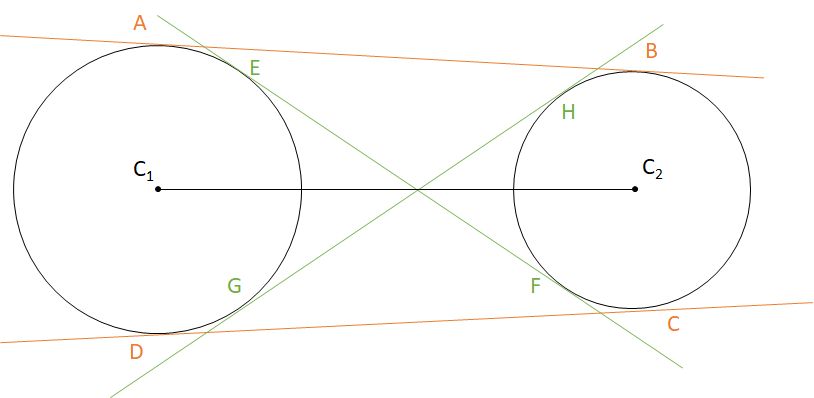​​​​​​​

Direct Common Tangent is always longer than Transverse Common Tangent

When two circles touch externally, the length of DCT = 2$\sqrt{{r}_{1}{r}_{2}}$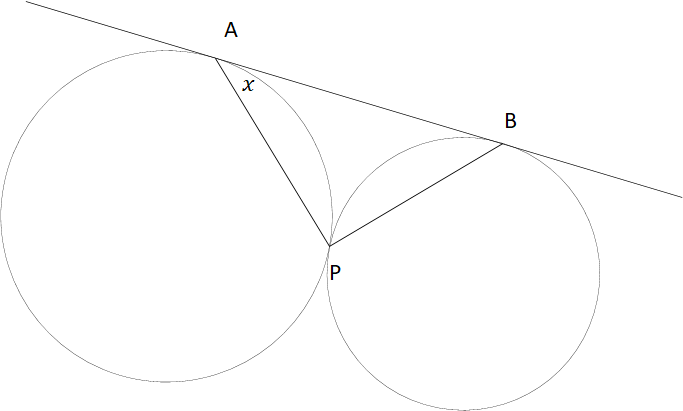​​​​​​​

## Feedback

Help us build a Free and Comprehensive Preparation portal for various competitive exams by providing us your valuable feedback about Apti4All and how it can be improved.